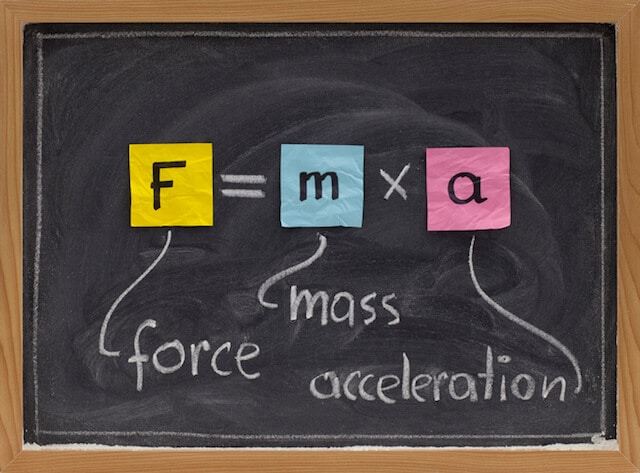Forces are all around us. Without them, you would not be able to pick up a pen, type on a keyboard, or even walk. As such, studying forces is essential to our understanding of the universe, and the subject of physics. This field of study is called dynamics – the study of motion under the action of forces.

A force is a push or pull on an object that causes its motion to change, if unopposed. They are the result of interactions between objects, and cannot exist without this interaction. Forces are vector quantities, meaning that they must have both magnitude and a direction. Some examples of forces are gravity, tension, and friction.

It is vital that you do not confuse ‘interaction’ and ‘contact’, a distinction which we will make clear in the subsequent sections. There are two main types of forces, contact and action-at-a-distance forces.

Action-at-a-distance forces

Action-at-a-distance forces can occur even when the objects are not physically in contact with each other. These include electrical, magnetic, and most prominently, gravitational forces.

Gravitational force, also known as weight, is the force with which objects with mass are attracted to one another. It is because of the Sun’s gravitational pull that the planets of the solar system are stuck orbiting around it, despite not being anywhere close to it. The same goes for the Moon’s orbit around the Earth, caused by the Earth’s gravity.

On Earth, all objects experience the planet’s gravitational pull, directed towards the centre of the Earth. This is why you do not float into space every time you jump – the Earth’s gravity pulls you back down.

Contact forces

Contact forces are what most people understand forces to be. They occur when two objects physically come into contact with each other. Here are some of the most prominent contact forces that you may encounter.

 Force Explanation Normal Normal force is the contact force that is perpendicular to the surface that the object contacts. You can think of this as a ‘support’ force. For example, consider a box placed on a table. The table exerts an upward force perpendicular to its surface, supporting the weight of the box. In this case, the normal force is equal to the weight (gravitational force) of the box, but in the opposite direction. Tension Tension force is the force that a string, rope, or wire exerts when it is pulled taut by forces acting from opposite ends. Tension is always directed parallel to the string, pulling equally on the objects at either end. Friction Friction is a force that is exerted on an object as it tries to move across a surface. Friction opposes motion and always acts parallel to the surface of contact. This means that friction acts in the direction directly opposite of the object’s movement. Friction occurs due to intermolecular forces of attraction between the object and surface, which hinders the movement of the object. Air resistance Air resistance is a subset of frictional force that acts on objects travelling through the air. Like friction, it acts in opposition to the movement of an object. Its magnitude and effect increase with the object’s surface area and speed, making it significant for objects like race cars or parachutes. However, on small scales, its magnitude is usually negligible and difficult to predict.

Free-body diagram

When multiple forces are acting on an object, it can be presented on a two-dimensional space as a free-body diagram. Free-body diagrams show the relative magnitude and direction of all the forces acting on a particular object at a given time.Before drawing a free-body diagram, you first have to identify the forces acting on an object in the first place. For this reason, it is imperative that you have a strong grasp on the different types of forces. In the case of the box sliding down a slope in the diagram, we have identified gravitational force, normal force, and friction as the forces acting on the box.

The directions of the arrows represent the directions of the forces relative to one another. The length of each arrow is also proportionate to the magnitude of each force, meaning a larger force would be represented by a longer arrow. These traits make a free-body diagram a concise way of presenting the most crucial information regarding the forces acting on an object.

Understanding the individual forces, their effects, and how to represent them is critical when dealing with the study of dynamics. The topic becomes even more complex when you need to consider how these forces interact and determine the resultant force.

These concepts can be challenging to grasp, so there is no shame in asking for help. You can engage the help of a qualified physics tuition centre to aid in your learning of these physics concepts. Here at Best Physics Tuition, our specially designed curriculum can not only give you a deeper understanding of the subject but also let you have fun while doing so.